DPL 9 Help

Laplace DistributionLaplace Distribution

Also known as the Double Exponential Distribution, the Laplace Distribution can appear to be a combination of the Triangular and Normal Distributions. The Laplace Distribution does not commonly apply to a wide range of cases, and care must be taken to ensure that the circumstances are appropriate when choosing the Laplace Distribution.

The Laplace Distribution is defined for all values of x, positive and negative. It has two parameters, a and b. The first parameter, a, can have any value, but the second parameter, b, must be positive. The distribution is: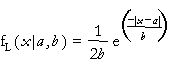where b>0. (x and a can have any value.)

The equations for the mean and variance are: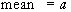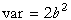The equations for the parameters are: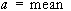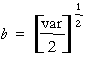Versions: DPL Professional, DPL Enterprise, DPL Portfolio